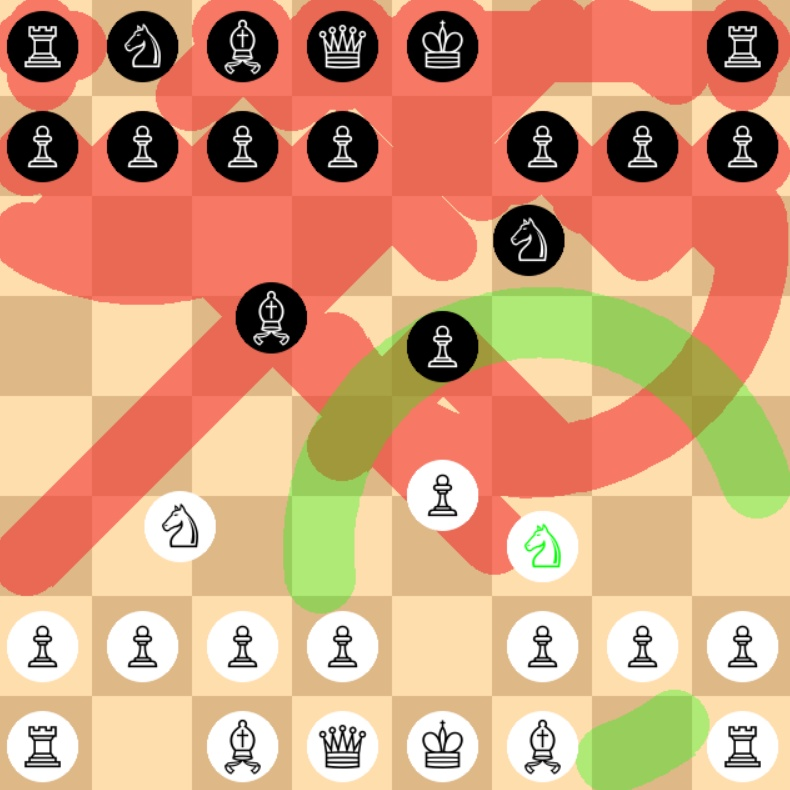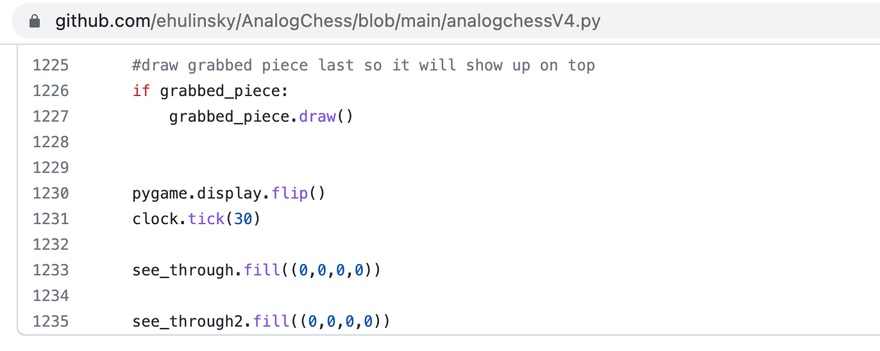# 用 Python + PyGame 编写国际象棋游戏``````import pygame
import math
from pygame import gfxdraw

def draw_circle(surface, x, y, radius, color):

def draw_circle_outline(surface, x, y, radius, color):

def getpolygon(origin, radius, N, start=0, end=None):
out = []
x, y = origin
Nf = float(N)
if end is None:
end = TAU
for i in range(N):
xp = x + radius * math.sin(end * i / Nf + start)
yp = y - radius * math.cos(end * i / Nf + start)
out.append((xp, yp))
return
``````https://github.com/ehulinsky/AnalogChess

0